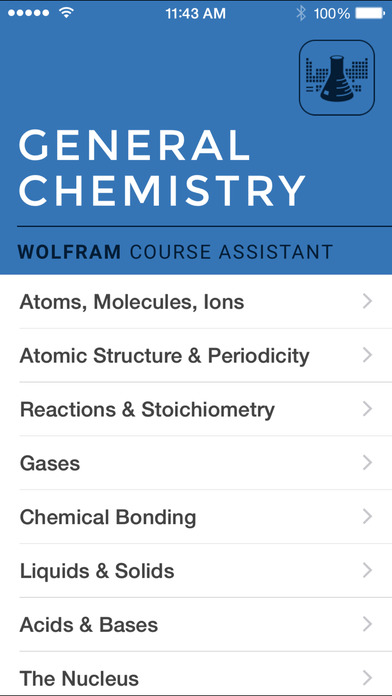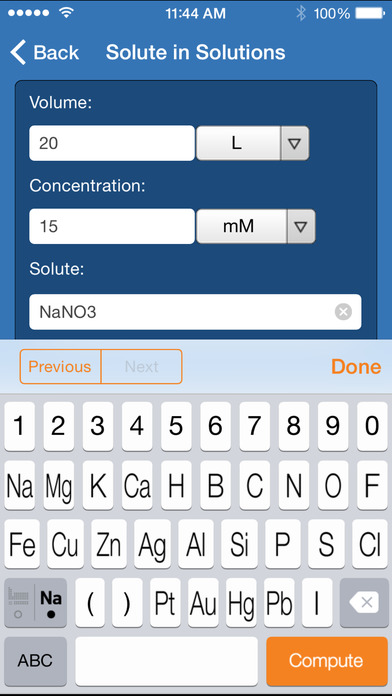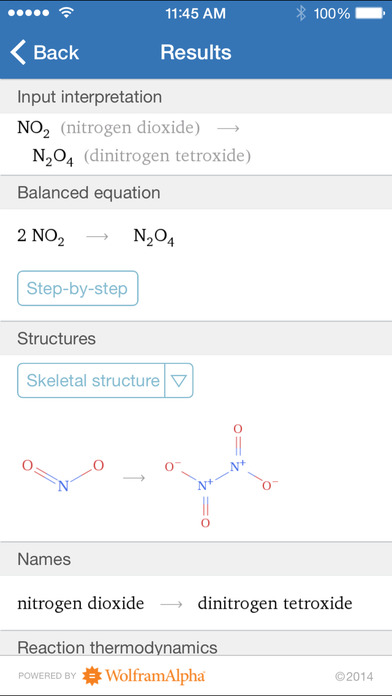# Wolfram General Chemistry Course Assistant

## iOS UniversalEducation

\$4.99

Taking introductory chemistry, or need a quick chemistry reference? Then you need the Wolfram General Chemistry Course Assistant. This comprehensive app for chemistry will help you quickly solve your homework problems, ace your tests, and quiz your understanding of basic chemistry concepts so you're prepared for your courses. Forget canned examples! The Wolfram General Chemistry Course Assistant solves your specific chemistry problems on the fly.

This app covers the following topics applicable to Introductory Chemistry, General Chemistry, and AP Chemistry:

- Determine properties of elements, chemicals, and ions
- Compute properties of gases using various gas laws
- Perform stoichiometric conversions for solutions
- Balance equations
- Compute the enthalpy, equilibrium constant, and theoretical yield of a reaction
- Perform over 45 different computations

The Wolfram General Chemistry Course Assistant is powered by the Wolfram|Alpha computational knowledge engine and is created by Wolfram Research, makers of Mathematica—the world's leading software system for mathematical research and education.

The Wolfram General Chemistry Course Assistant draws on the computational power of Wolfram|Alpha's supercomputers over a 3G, 4G, or Wi-Fi connection.

## What's New

- Bug fixes

•••••